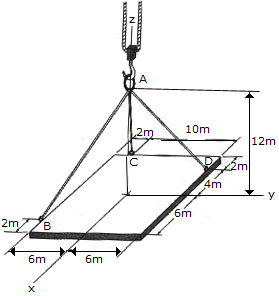# Engineering Mechanics - Equilibrium of a Particle - Discussion

### Discussion :: Equilibrium of a Particle - General Questions (Q.No.10)

10.The ends of the three cables are attached to ring at A and to the edge of a uniform 150-kg plate. Determine the tension in each of the cables for equilibrium.

 [A]. Fab = 629 N, Fac = 1030N, Fad = 57.0 N [B]. Fab = 1116 N, Fac = 1030N, Fad = 429 N [C]. Fab = 720 N, Fac = 116.1N, Fad = 830 N [D]. Fab = 858 N, Fac = 0, Fad = 858 N

Explanation:

No answer description available for this question.

 Manivannan said: (Sep 8, 2015) I need explanation for this question.